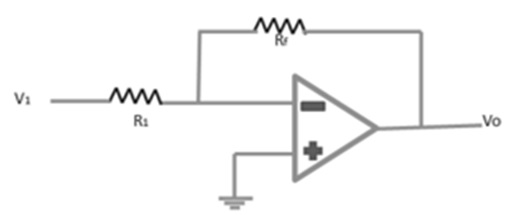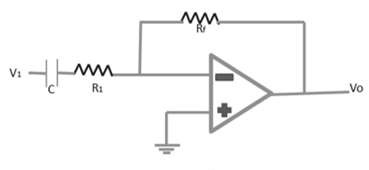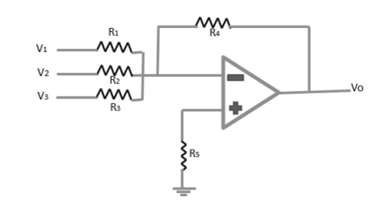# Semiconductor Devices - Practical Op-Amps

## Inverting Amplifier

The following figure shows an inverting amplifier. The input signal is amplified and inverted. This is the most widely used constant-gain amplifier circuit.

Vo = -Rf.Vin /R1

Voltage gain A = (-Rf /R1)## Non-Inverting Amplifier

The following figure shows an op-amp circuit that works as a non-inverting amplifier or constant-gain multiplier and it has better frequency stability.

The input signal is amplified but it is not inverted.

Output Vo = [(R1 + Rf) / R1] V1

Voltage gain A = (R1 + Rf) / R1## Inverting Summing Amplifier

The following figure shows an inverting summing amplifier. It is the most used circuit of the op-amp. The circuit shows a three-input summing amplifier, which provides a means of algebraically summing three voltages, each multiplied by a constant-gain factor. The output voltage is expressed as,

Vo = [(-R4 / R1) V1][(-R4 / R2) V2][(-R4 / R3) V3]

Vo = -R4(V1 / R1 + V2 / R2 + V3 / R3)

If, R1 = R2 = R3 = R4 = R & Rs = R/3

Vo = -(V1 + V2 + V3)